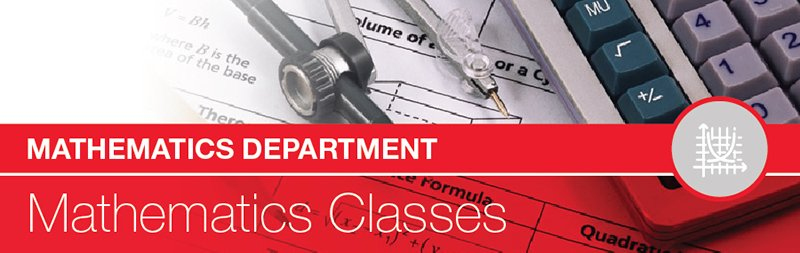The mathematics curriculum is designed to accommodate students whose interests lie in mathematics, as well as students whose interests lie in other areas of study. Our courses are designed to engage students in the practice of mathematics by developing an understanding of mathematical relationships and mathematical models both in and out of context with an emphasis on problem solving and reasoning. Topics will be developed and valued conceptually leading to procedural fluency. A variety of instructional techniques and technologies will be implemented by teachers.
Any six semesters of mathematics, successfully completed, fulfill the three mathematics credit requirement for high school graduation. Students must complete Algebra 1 and a mathematics course that includes geometry content in order to fulfill the mathematics requirements. Most colleges require students to complete a minimum of 3 years of math: Algebra 1, Geometry, and Algebra 2.
For those courses requiring a graphing calculator, the Math Department recommends the Texas Instrument – 84 Plus (TI – 84 Plus) model.

MATHEMATICS COURSE OFFERINGS

Introduction to Algebra X X X Department Placement Required
Survey of Math Topics X X X X Department Placement Required
Algebra 1 X X X None
Blended Algebra 1 X X X None
Algebra 1 Support X X X Department Placement Required
Algebra 1 w/ Geometry X Department Placement Required
Geometry Core X X X Department Placement Required
Geometry X X X Algebra 1
Blended Geometry X X X Algebra 1
Online Geometry X X X Algebra 1
Honors Geometryw X X Algebra 1 and Department Placement
Algebra 2 Core X X Department Placement Required
Algebra 2 X X X Geometry
Blended Algebra 2 X X X Geometry
Online Algebra 2 X X X Geometry
Honors Algebra 2w X X X Honors Geometry
Quantitative Literacy and Statistics X Algebra 2 Core
Advanced Placement Statisticsw X X X Algebra 2 or Honors Algebra 2
Business Precalculus X X Algebra 2 or Algebra 2 Core
Blended Business Precalculus X X Algebra 2 or Algebra 2 Core
Precalculus X X Algebra 2 or Honors Algebra 2
Blended Precalculus X X Algebra 2 or Honors Algebra 2
Honors Precalculusw X X X Honors Algebra 2
Advanced Placement Calculus ABw X X Precalculus or Honors Precalculus
Advanced Placement Calculus BC w X X X Honors Precalculus
Multivariable Calculusw (NCHS Only) X X AP Calculus BC
Multivariable Calculus w/Lin Algebra w (NNHS Only) X X AP Calculus BC
STEM Capstone-Blended Learning X X Instructional Coordinator Approval

w = weighted course

RECOMMENDED SEQUENCES OF CLASSES:

FRESHMEN SOPHOMORE JUNIOR SENIOR
Honors Geometry Honors Algebra 2 Honors Pre-Calculus and/or AP Statistics A.P. Calculus “BC” and/or A.P. Statistics
Geometry OR Algebra 1 with Geometry Algebra 2 Pre-Calculus and/or AP Statistics A.P. Calculus “AB” and/or A.P. Statistics
Algebra 1 Geometry Algebra 2 Business Pre-Calculus, Pre-Calculus and/or A.P. Statistics, or Quantitative Literacy and Statistics
Introduction to Algebra Algebra 1 Geometry or Geometry Core Algebra 2 or Algebra 2 Core

DEPARTMENT PLACEMENT:

For incoming Freshmen: Students will be placed by the Mathematics Department based on their 8th Grade math course, math grades, and recommendations from the junior high teachers and counselors.

For those entering Sophomore, Junior, or Senior year: Students will be placed according to the following recommended sequence of classes. To be considered for a change to an honors course, a student’s average in the previous course must be a strong “A”. A student must also have the math teacher’s recommendation. Exceptions to the above will be considered by the Math Instructional Coordinator.